# Electric Current, Electric Potential & Potential Difference Notes | Study Science Class 10 - Class 10

## Class 10: Electric Current, Electric Potential & Potential Difference Notes | Study Science Class 10 - Class 10

The document Electric Current, Electric Potential & Potential Difference Notes | Study Science Class 10 - Class 10 is a part of the Class 10 Course Science Class 10.
All you need of Class 10 at this link: Class 10

Electricity

The source of all electricity is in charge. As the charge is the basis of all electrical phenomena, we need to know the amount of charge on a body. It is measured in coulombs. The coulomb is the SI unit of charge, and its symbol is C.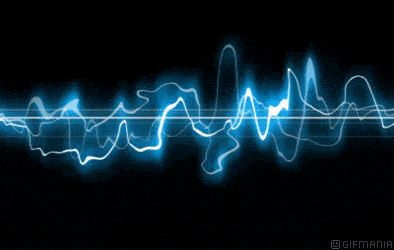Electricity passing through a wire

• The matter is generally made of protons, electrons and neutrons. Each proton carries a charge of 1.6 × 10-19 coulomb, and each electron carries an equal negative charge.
• Neutrons do not carry any net charge. Normally, a body has equal number of protons and electrons, and is, therefore, electrically neutral.
• In certain situations, the balance of charges in a body is disturbed.
Example: When a glass rod is rubbed with a silk cloth, some electrons get transferred from the glass rod to the silk. The silk cloth, which gains electrons, becomes negatively charged. And the glass rod, which is left with more protons than electrons, becomes positively charged.
• Charged particles or objects can exert forces on each other.
• Unlike charges attract each other.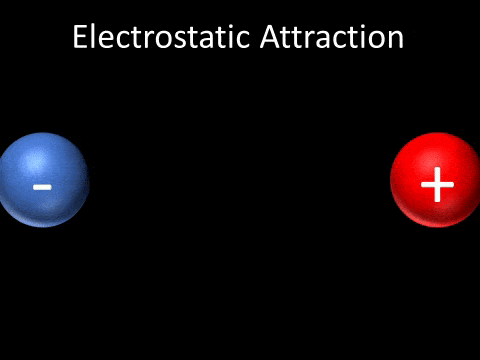• Like (similar) charges repel each other.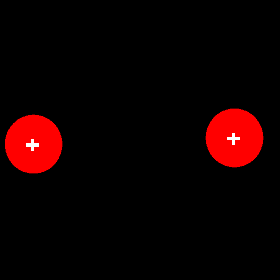Electrostatic Repulsion
• Another important thing about charged particles is that they can flow, i.e., they can move in a particular direction. This flow of charged particles is called an electric current. Charged particles such as electrons are present in all substances. But they do not flow on their own. For the flow of charges, there has to be a potential difference.

Potential Difference and the Flow of Charge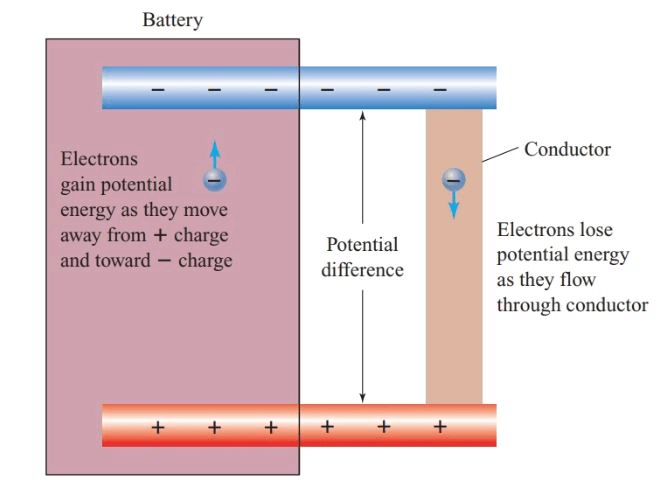• The potential difference between two points A and B is the work done per unit charge in taking charge from B to A.
• We express this mathematically as: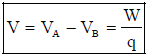• Here, V is the potential difference between the points A and B, and VA and VB are the potentials at these points. The potential at infinity is chosen as zero.
• If B is the reference point, the potential at B is VB = O.
• From Equation, the potential at A is VA = W/q. So, the potential at a point is the work done per unit charge in taking charge to that point from a chosen reference point.
The equation may also be written as:
W= qV
• The work done on the charge q is stored as the electric potential energy (U) of the group of charges.
U = qV
• Unit of potential difference
The unit of potential difference (and potential) is the volt, whose symbol is V. One volt is the potential difference between two points in a current-carrying conductor when 1 joule of work is done to move a charge of 1 coulomb from one point to the other.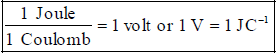• The potential difference between two points is sometimes also called the voltage.
• Flow of Charge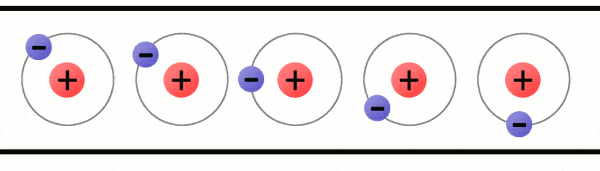Flow of Charge

• Consider two identical metallic spheres P and N, carrying equal amounts of positive and negative charges, respectively. A positive charge is to be taken from B to A. It is attracted by the negatively charged sphere N and repelled by the positively charged sphere P. So, to move the charge towards A, one has to apply a force on it towards the left. Thus, the work done is positive. Hence, the potential difference VA - V is positive. This means VA > VB
• As one move towards P, the workdone increases; so, the potential increases. And on moving towards N, the potential decreases. So, the potential of P is higher than that of N.

In general, the potential of a positively charged body is taken as higher than that of a negatively charged body.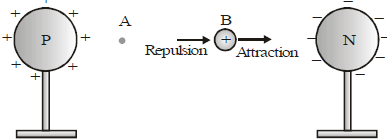What happens when a free-to-move charge is placed between the spheres?

A positive charge will move towards the negatively charged sphere. And a negative charge will move towards the positively charged sphere. That is, a free positive charge moves towards lower potential. And a free negative charge moves towards higher potential.

If the two spheres are connected by a metal wire, electrons from the negatively charged sphere (at a lower potential) will flow to the positively charged sphere (at a higher potential). Eventually, the flow of electrons causes the charges on the spheres to become balanced. When that happens, the spheres no longer carry a net charge, and therefore, have equal potential. So, the flow of electrons stops. So we can say that a potential difference causes charges to flow.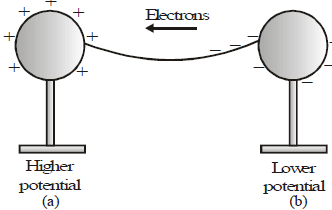A Cell Provides a Constant Potential Difference

• The potential difference provided by things like-charged spheres reduces to zero quickly once charges start to flow. So, we have to use cells to provide the constant potential difference for a long time.
• Cells have chemicals inside. Reactions in the cell cause positive and negative charges to gather separately. This creates a potential difference between the terminals of the cell.
• The terminal at higher potential is called the positive terminal, and the one at lower potential is called the negative terminal.
• The cells that we commonly use are called dry cells (fig a). In a common dry cell, the small metallic cap at one end is the positive terminal, while the flat metallic plate at the other end is the negative terminal. It provides a potential difference of 1.5 V.
• A cell is represented by the symbol shown in fig (b). The larger line represents the positive terminal, while the shorter line represents the negative terminal.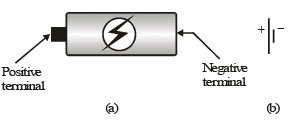Dry cell and symbol of a cell

A combination of cells is called a battery.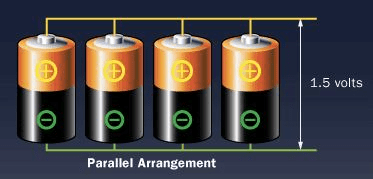Battery

• Quite often, multiple cells are combined to get a higher potential difference than that of a single cell.
Example: We connect two 1.5V cells to get a potential difference of 3V (Figure (c)) This is shown using symbols in Figure (d).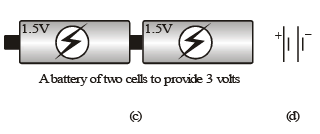Electric Current

• Consider a metallic wire ACB connected across a cell of potential difference V. Since the end A is connected to the positive terminal, it is at a higher potential than the end B.
• In metals, some electrons are loosely bound to the atoms and can move within it. These are called free electrons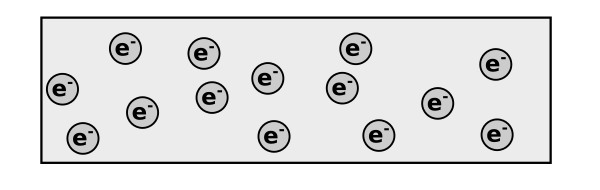Free electrons
• In the metallic wire, these electrons (negative charges) move from the low-potential side B to the high-potential side A.
• After reaching A, they enter the cell. The chemical reactions in the cell drive these electrons to the negative terminal. From there, they re-enter the wire at the end B. Thus, there is a continuous flow of electrons in the wire from B to C to A.
• We say that there is an electric current in the wire. In a metal, the flow of negative charges constitutes the current.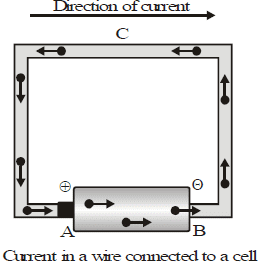• An electric current can also be a flow of positive charges. So, a flow of charge is called an electric current.
• By convention, the direction of current is taken as the direction of flow of positive charges. Thus, the direction of the current is opposite to the direction of flow of negative charges.
• So, when a wire is connected to a cell, the current in the wire is from the positive-terminal end to the negative terminal end.

Measurement of Current

• The charge passing per unit time through a given place(area) is the magnitude of the electric current at that place. Thus,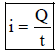• Here Q is the charge that passes through a place in time t.
• Unit of current from Equation, we find that current is charge divided by time. The SI unit of charge is the coulomb and that of time is the second. The SI unit of current, therefore, is coulomb/second. This unit is called the ampere, whose symbol is A. Thus if one coulomb of charge passes through a place in one second, the current there is 1 ampere.

Conductors and Insulators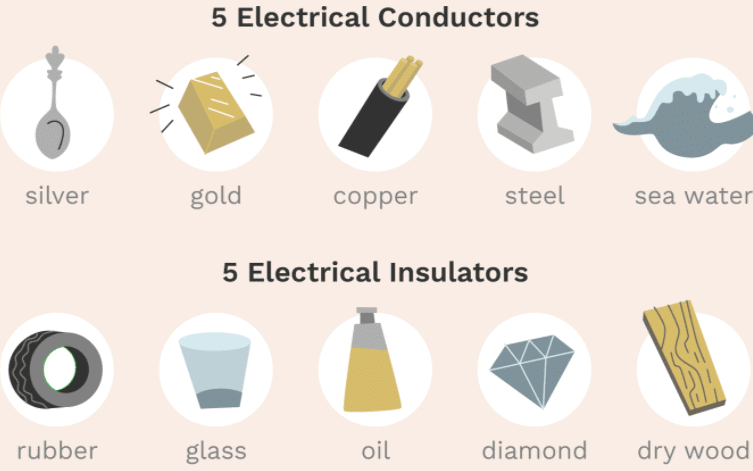• Materials that conduct electricity easily are called good conductors or simply, conductors. And, materials that do not conduct electricity easily are called insulators.
• All metals conduct electricity because they have some loosely bound free electrons, which flow when a potential difference is applied. However, some metals conduct electricity better than others. Silver is the best conductor. But because of the high cost of silver, electric wires are made of copper, or in some cases aluminium.
• Most nonmetallic solids do not conduct electricity. Although diamond and graphite are both forms of carbon (a nonmetal), graphite is a conductor while diamond is an insulator.
• Insulators do not conduct electricity because their electrons are tightly bound to the atoms. Rubber, plastics, wood, glass and porcelain are some examples of insulators. Insulators have many uses. For example, they are used as protective covers on electric wires and electrician's tools.
• Certain liquids also conduct electricity. While distilled water is an insulator, the addition of certain salts, acids or bases allows it to conduct electricity. Under normal circumstances, gases do not conduct electricity.
The document Electric Current, Electric Potential & Potential Difference Notes | Study Science Class 10 - Class 10 is a part of the Class 10 Course Science Class 10.
All you need of Class 10 at this link: Class 10Use Code STAYHOME200 and get INR 200 additional OFF

## Science Class 10

107 videos|325 docs|162 tests

Track your progress, build streaks, highlight & save important lessons and more!

,

,

,

,

,

,

,

,

,

,

,

,

,

,

,

,

,

,

,

,

,

,

,

,

;